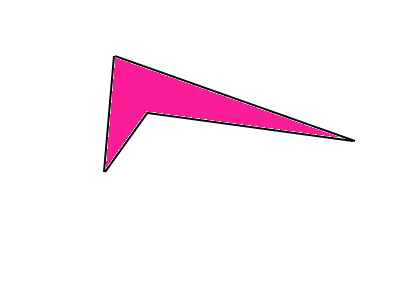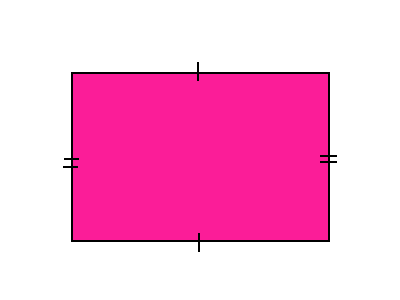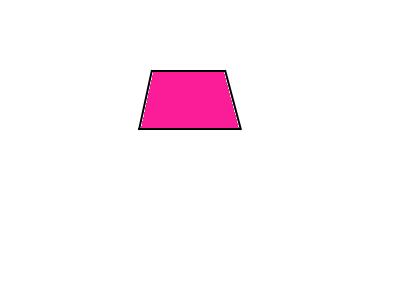# Common Core: 3rd Grade Math : Reason with Shapes and their Attributes

## Example Questions

← Previous 1 3 4

### Example Question #1 : Understand Shape Categories: Ccss.Math.Content.3.G.A.1

The shape below can be categorized as a ____________.Possible Answers:

rectangle

none of the choices

rhombus

square

quadrilateral

Correct answer:

quadrilateral

Explanation:

This shape is a quadrilateral because it hassides, and by definition a-sided shape is a quadrilateral.

This shape cannot be a square because it does not haveequal side lengths.

This shape cannot be a rectangle because its opposite sides are not equal in length, nor are the angles alls.

This shape cannot be a rhombus because it does not have opposite, parallel sides and angles, nor does it have equal side lengths.

### Example Question #1 : Reason With Shapes And Their Attributes

The shape below can be categorized as a ____________.Possible Answers:

rectangle, parallelogram, and rhombus

rectangle, parallelogram, and triangle

rectangle, parallelogram, and trapezoid

rectangle, parallelogram, and quadrilateral

rectangle, square, and quadrilateral

Correct answer:

rectangle, parallelogram, and quadrilateral

Explanation:

This shape is a rectangle because a rectangle has to have allangles, and a this shape has allangles. But, a rectangle is also a quadrilateral and a parallelogram.

A rectangle can be categorized as a quadrilateral because by definition a quadrilateral is a-sided figure, and a rectangle hassides.

A rectangle can be categorized as a parallelogram because a parallelogram has to havesides with opposite sides being parallel, and a rectangle hassides with opposite sides being parallel.

### Example Question #3 : Reason With Shapes And Their Attributes

The shape below can be categorized as a ____________.Possible Answers:

parallelogram and a square

trapezoid and a quadrilateral

trapezoid and a parallelogram

parallelogram and a rhombus

trapezoid and a square

Correct answer:

trapezoid and a quadrilateral

Explanation:

This shape is a trapezoid because a trapezoid only has one set of parallel sides, which in this case is the top and the base lines.

This shape is also a quadrilateral because it hassides, and a quadrilateral is any-sided figure.

This shape is not a parallelogram because a parallelogram has two sets of parallel sides, and this shape only has one.

This shape is not a rhombus because a rhombus has to haveequal sides, or opposite equal acute angles, and this shape does not haveequal sides, nor are the opposite angles equal or acute. The two top angles are obtuse angles.

### Example Question #2 : Reason With Shapes And Their Attributes

Can a triangle be called a quadrilateral?

Possible Answers:

No

Yes

Correct answer:

No

Explanation:

A triangle cannot be called a quadrilateral because a quadrilateral is a-sided figure, and a triangle only hassides.

### Example Question #4 : Reason With Shapes And Their Attributes

Can a square be called a quadrilateral?

Possible Answers:

Yes

No

Correct answer:

Yes

Explanation:

A square can be called a quadrilateral because quadrilaterals are any-sided shape, and a square hassides.

### Example Question #2 : Reason With Shapes And Their Attributes

Can a square be called a rectangle?

Possible Answers:

No

Yes

Correct answer:

Yes

Explanation:

A square can be called a rectangle because it has two sets of equal sides and a square has allangles.

### Example Question #3 : Understand Shape Categories: Ccss.Math.Content.3.G.A.1

Can a pentagon be classified as a quadrilateral?

Possible Answers:

No

Yes

Correct answer:

No

Explanation:

A pentagon cannot be classified as a quadrilateral because a pentagon hassides, and a quadrilateral is a-sided figure.

### Example Question #7 : Reason With Shapes And Their Attributes

Can a square be called a parallelogram?

Possible Answers:

No

Yes

Correct answer:

Yes

Explanation:

A square can be called a parallelogram because a parallelogram has to have two sets of parallel sides, and a square has two lets of parallel sides.

### Example Question #4 : Reason With Shapes And Their Attributes

Can a rectangle be called a square?

Possible Answers:

Yes

No

Correct answer:

No

Explanation:

A rectangle cannot because called a square because a square has to haveequal sides and a rectangle does not haveequal sides, it hassets of equal sides.

### Example Question #4 : Understand Shape Categories: Ccss.Math.Content.3.G.A.1

Can a trapezoid be categorized as a square?

Possible Answers:

No

Yes

Correct answer:

No

Explanation:

A trapezoid cannot be categorized as a square because a square has to haveequal sides, and a trapezoid does not haveequal sides.

← Previous 1 3 4

### All Common Core: 3rd Grade Math Resources